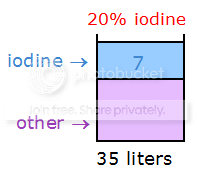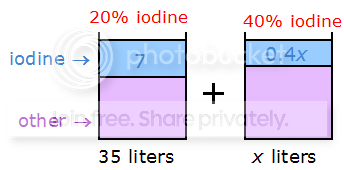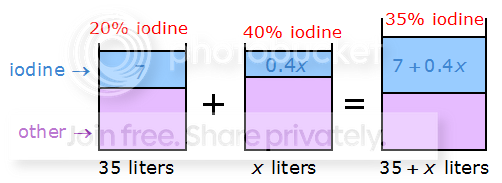GMAT Question of the Day - Daily to your Mailbox; hard ones only

 It is currently 16 Jun 2019, 22:36GMAT Club Daily Prep

Thank you for using the timer - this advanced tool can estimate your performance and suggest more practice questions. We have subscribed you to Daily Prep Questions via email.

Customized
for You

we will pick new questions that match your level based on your Timer History

Track

every week, we’ll send you an estimated GMAT score based on your performance

Practice
Pays

we will pick new questions that match your level based on your Timer History

Not interested in getting valuable practice questions and articles delivered to your email? No problem, unsubscribe here.How many liters of a 40% iodine solution need to be mixed with 35 lite

Author Message
TAGS:

Hide Tags

Math ExpertV
Joined: 02 Sep 2009
Posts: 55618
How many liters of a 40% iodine solution need to be mixed with 35 lite  [#permalink]

Show Tags

1
900:00

Difficulty:15% (low)

Question Stats:79% (01:52) correct21% (02:23) wrongbased on 235 sessions

HideShow timer Statistics

How many liters of a 40% iodine solution need to be mixed with 35 liters of a 20% iodine solution to create a 35% iodine solution?

A. 35
B. 49
C. 100
D. 105
E. 140

_________________
CEOV
Joined: 12 Sep 2015
Posts: 3778
Re: How many liters of a 40% iodine solution need to be mixed with 35 lite  [#permalink]

Show Tags

3
Top Contributor
2
Bunuel wrote:
How many liters of a 40% iodine solution need to be mixed with 35 liters of a 20% iodine solution to create a 35% iodine solution?

A. 35
B. 49
C. 100
D. 105
E. 140

One approach is to sketch the solutions with their components separated.20% of 35 liters = 7 liters, so the original solution contains 7 liters of iodine.

To this solution, we'll add x liters of the solution that's 40% iodine.40% of x liters = 0.4x liters. So, we're adding 0.4x liters of iodine.

When we combine the two solutions, it's easy to see how the resulting mixture looks, since we need only add the volumes of iodine in each solution.As you can see, our resulting mixture has a TOTAL volume of 35 + x liters.
We can also see that the resulting mixture contains 7 + 0.4x liters of iodine.
Since the resulting mixture must by 35% (aka 35/100) iodine, we can write the following equation:
(7 + 0.4x)/(35 + x liters) = 35/100
First simplify 35/100 to get: (7 + 0.4x)/(35 + x liters) = 7/20
Cross multiply to get: 20(7 + 0.4x) = 7(35 + x liters)
Expand: 140 + 8x = 245 + 7x
Solve: x = 105

RELATED VIDEO

_________________
Math ExpertV
Joined: 02 Aug 2009
Posts: 7742
Re: How many liters of a 40% iodine solution need to be mixed with 35 lite  [#permalink]

Show Tags

3
1
Bunuel wrote:
How many liters of a 40% iodine solution need to be mixed with 35 liters of a 20% iodine solution to create a 35% iodine solution?

A. 35
B. 49
C. 100
D. 105
E. 140

These Qs are best done with weighted Average method....

Two solutions - x of 40% and 35 of 20% results in a solution of 35% solution..

SO ratio = $$\frac{QTY- of- 40}{Qty- of- 20%} =\frac{35-20}{40-35} = \frac{15}{5}.....$$
so $$\frac{x}{35} = \frac{15}{5} = 3..................x = 35*3 = 105$$

D
_________________
General Discussion
SVPB
Joined: 06 Nov 2014
Posts: 1877
Re: How many liters of a 40% iodine solution need to be mixed with 35 lite  [#permalink]

Show Tags

1
4
Bunuel wrote:
How many liters of a 40% iodine solution need to be mixed with 35 liters of a 20% iodine solution to create a 35% iodine solution?

A. 35
B. 49
C. 100
D. 105
E. 140

Solution 1:
Assume the iodine solution to be mixed = x lts.
Iodine = 0.4x lts, Water = 0.6x lts.

Solution 2: 35 liters of a 20% iodine solution
Iodine = 7 lts, Water = 28 lts.

Total iodine = 0.4x + 7
Total water = 0.6x + 28

The resultant is a 35% idoine solution.
Hence (0.4x + 7) / (x + 35) = 35/100
40x + 700 = 35x + 1225
5x = 525
x = 105 lts

Correct Option: D
Board of DirectorsD
Joined: 01 Sep 2010
Posts: 3360
Re: How many liters of a 40% iodine solution need to be mixed with 35 lite  [#permalink]

Show Tags

1
2
A more straight approach

$$0.4X + 0.2 Y = 0.35X + 0.35 Y$$

$$0.05X = 0.15Y$$ AND our $$Y = 35$$

$$0.05X = 5.25$$

$$X = \frac{5.25}{0.05} = 105$$

D is the one
_________________
Current StudentJoined: 18 Oct 2014
Posts: 831
Location: United States
GMAT 1: 660 Q49 V31GPA: 3.98
Re: How many liters of a 40% iodine solution need to be mixed with 35 lite  [#permalink]

Show Tags

1
Bunuel wrote:
How many liters of a 40% iodine solution need to be mixed with 35 liters of a 20% iodine solution to create a 35% iodine solution?

A. 35
B. 49
C. 100
D. 105
E. 140

60% water solution mixed with 80% water solution to get 65% water solution.

Solution will be mixed in the ratio::-

x/35= 80-65/65-60
x/35= 15/5
x= 3*35= 105

_________________
I welcome critical analysis of my post!! That will help me reach 700+
InternB
Joined: 12 Aug 2013
Posts: 46
Re: How many liters of a 40% iodine solution need to be mixed with 35 lite  [#permalink]

Show Tags

D it will be

weighted avg concept :-

20 --------------------35-------------------40

qyantity of 20%/quantity of 40% = 40- 35/35-20

35/x = 5/15
x = 105
DirectorS
Joined: 21 Mar 2016
Posts: 518
Re: How many liters of a 40% iodine solution need to be mixed with 35 lite  [#permalink]

Show Tags

Bunuel wrote:
How many liters of a 40% iodine solution need to be mixed with 35 liters of a 20% iodine solution to create a 35% iodine solution?

A. 35
B. 49
C. 100
D. 105
E. 140

60% water solution mixed with 80% water solution to get 65% water solution.

Solution will be mixed in the ratio::-

x/35= 80-65/65-60
x/35= 15/5
x= 3*35= 105

Hi, Divyadisha ,, may i knw how did u get that formula
DirectorS
Joined: 21 Mar 2016
Posts: 518
Re: How many liters of a 40% iodine solution need to be mixed with 35 lite  [#permalink]

Show Tags

chetan2u wrote:
Bunuel wrote:
How many liters of a 40% iodine solution need to be mixed with 35 liters of a 20% iodine solution to create a 35% iodine solution?

A. 35
B. 49
C. 100
D. 105
E. 140

These Qs are best done with weighted Average method....

Two solutions - x of 40% and 35 of 20% results in a solution of 35% solution..

SO ratio = $$\frac{QTY- of- 40}{Qty- of- 20%} =\frac{35-20}{40-35} = \frac{15}{5}.....$$
so $$\frac{x}{35} = \frac{15}{5} = 3..................x = 35*3 = 105$$

D

hi chetan2u can u plz explain tat formula,
ManagerB
Joined: 16 Mar 2016
Posts: 126
Location: France
GMAT 1: 660 Q47 V33GPA: 3.25
Re: How many liters of a 40% iodine solution need to be mixed with 35 lite  [#permalink]

Show Tags

If x is the number of liter of the 40% iodine solution, we have :
0.4 *x + 0.2 * 35 = 0.35 ( 35 + x)
0.4 *x + 0.2 * 35 = 0.35 * 35 + 0.35 * x
0.05 * x = 35 (0.35 - 0.2)
0.05 * x = 35 * 0.15
x = 35 * 3 = 105
VPP
Joined: 07 Dec 2014
Posts: 1199
Re: How many liters of a 40% iodine solution need to be mixed with 35 lite  [#permalink]

Show Tags

let x=liters of 40% iodine solution needed
.4x+(.2)(35)=.35(x+35)
x=105 liters
Board of DirectorsP
Status: QA & VA Forum Moderator
Joined: 11 Jun 2011
Posts: 4501
Location: India
GPA: 3.5
Re: How many liters of a 40% iodine solution need to be mixed with 35 lite  [#permalink]

Show Tags

Bunuel wrote:
How many liters of a 40% iodine solution need to be mixed with 35 liters of a 20% iodine solution to create a 35% iodine solution?

A. 35
B. 49
C. 100
D. 105
E. 140

Attachment:Capture.PNG [ 4.45 KiB | Viewed 4906 times ]

35% ----> 7 + 0.4x
100%---->$$\frac{(7 + 0.4x)}{35} *100$$ = $$\frac{(7 + 0.4x)}{7}*20$$

Or, $$\frac{(140 + 8x)}{7}$$ = 35 + x

Or, 140 + 8x = 245 + 7x

Or, x = 245 - 140

Or, x = 105

Hence answer will be (D) 105
_________________
Thanks and Regards

Abhishek....

PLEASE FOLLOW THE RULES FOR POSTING IN QA AND VA FORUM AND USE SEARCH FUNCTION BEFORE POSTING NEW QUESTIONS

How to use Search Function in GMAT Club | Rules for Posting in QA forum | Writing Mathematical Formulas |Rules for Posting in VA forum | Request Expert's Reply ( VA Forum Only )
CEOV
Joined: 12 Sep 2015
Posts: 3778
Re: How many liters of a 40% iodine solution need to be mixed with 35 lite  [#permalink]

Show Tags

2
Top Contributor
Bunuel wrote:
How many liters of a 40% iodine solution need to be mixed with 35 liters of a 20% iodine solution to create a 35% iodine solution?

A. 35
B. 49
C. 100
D. 105
E. 140

Another approach is to use Weighted Averages:

Weighted average of groups combined = (group A proportion)(group A average) + (group B proportion)(group B average) + (group C proportion)(group C average) + ...

In this case, we have:
35% iodine = (proportion of 20% solution)(20% iodine ) + (proportion of 40% solution)(40% iodine)

To determine the proportions, let's let x = the volume of 40% solution needed
We already know we have 35 liters of 20% solution
This means the TOTAL volume = 35 + x
So, the proportion of 20% solution 35/(35 + x)
And the proportion of 40% solution x/(35 + x)

Plug these values into formula to get: 35 = [35/(35 + x)](20) + [x/(35 + x)](40)
Multiply both sides by (35 + x) to get: 35(35 + x) = (35)(20) + (x)(40)
Expand: 1225 + 35x = 700 + 40x
Subtract 700 from both sides: 525 + 35x = 40x
Subtract 35x from both sides: 525 = 5x
Solve: x = 105

RELATED VIDEO

_________________
DirectorV
Joined: 27 May 2012
Posts: 800
How many liters of a 40% iodine solution need to be mixed with 35 lite  [#permalink]

Show Tags

1
Bunuel wrote:
How many liters of a 40% iodine solution need to be mixed with 35 liters of a 20% iodine solution to create a 35% iodine solution?

A. 35
B. 49
C. 100
D. 105
E. 140

Dear Moderator,
Please change tag from " work/rate" to "mixture Problems ". Thank you.
_________________
- Stne
Math ExpertV
Joined: 02 Sep 2009
Posts: 55618
Re: How many liters of a 40% iodine solution need to be mixed with 35 lite  [#permalink]

Show Tags

stne wrote:
Bunuel wrote:
How many liters of a 40% iodine solution need to be mixed with 35 liters of a 20% iodine solution to create a 35% iodine solution?

A. 35
B. 49
C. 100
D. 105
E. 140

Dear Moderator,
Please change tag from " work/rate" to "mixture Problems ". Thank you.

_________________
Done. Thank you.
_________________Re: How many liters of a 40% iodine solution need to be mixed with 35 lite   [#permalink] 15 Sep 2018, 05:31
Display posts from previous: Sort by

How many liters of a 40% iodine solution need to be mixed with 35 lite